# Analyzing Semiconductor Device Surfaces and Electrical Properties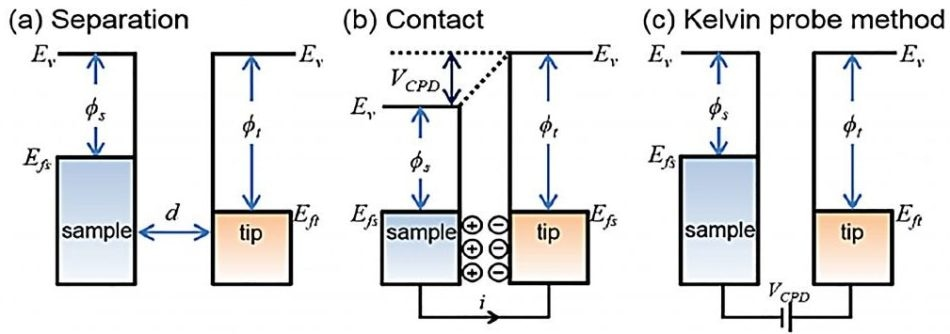Figure 1. Electronic energy levels of the sample and AFM tip for three cases: (a) tip and sample are separated by distance d with no electrical contact, (b) tip and sample are in electrical contact, and (c) external bias (VDC) is applied between tip and sample to nullify the CPD and, therefore, the tip–sample electrical force. Ev is the vacuum energy level. Efs and Eft are Fermi energy levels of the sample and tip, respectively.

## Using KPFM with Park AFM to Analyze Electrical Properties of Metal, Semiconductor Device Surfaces, Organic and Biological Materials

Kelvin probe force microscopy, or KPFM, is a tool that measures the potential difference in local contact between a conductive atomic force microscopy (AFM) tip and the sample. Therefore, it ensures high spatial resolution of the mapped work function or surface potential of the sample.

Since being first introduced by Nonnenmacher , KPFM has been widely used as a unique method to characterize the electrical properties of metal, semiconductor surfaces and semiconductor devices at nanoscale.

In recent years, SKRM has also been used to study the electrical properties of organic materials, devices [2–4], and biological materials. To make sure there is no confusion, the bullet points below show all of the synonyms of the technique:

• SKPM: Scanning Kelvin Probe Microscopy
• KPFM: Kelvin Probe Force Microscopy
• SSPM: Scanning Surface Potential Microscopy
• SKFM: Scanning Kelvin Force Microscopy
• SP-AFM: Surface Potential Atomic Force Microscope

### Microscopy

The following paper uses ‘KPFM’ because it is the most commonly-used descriptor for the microscopic technique. The technique also includes the term ’Kelvin force,’ which marks the similarities between the microscopic and macroscopic techniques with the Kelvin probe method. The measured value for both techniques is the same, even though the methodology is slightly different. To avoid confusion, this note refers only to the microscopic technique, KPFM.

### Fundamentals of KPFM

KPFM measures Contact Potential Difference (CPD) between a conductive AFM tip and a sample. The CPD (VCPD) between the tip and sample is defined as:

(2.1) where sample and tip are the work functions of the sample and tip, and e is the electronic charge. Electrical force, such that causes the AFM tip to be brought near the sample surface, is caused by the different Fermi energy levels between the AFM tip and sample surface.

Figure 1 presents a diagram of the tip and sample surface that shows the difference in energy level. Figure 1(a) shows what the energy levels are when then tip and sample surface are separated but are close enough for electron tunneling to be possible. Equilibrium of the states requires Fermi levels to line-up at a state of equilibrium. The Fermi levels align through the flow of electrons, but only upon electric contact, at which point, the system reaches an equilibrium state (as shown in Figure 1(b)). Thus, the tip and the sample surface are charged, and the apparent VCPD is formed. Even though the Fermi levels have aligned, the vacuum levels are now different.

Due to the VCPD, an electrical force acts on the contact area. This is presented in Figure 1(c). It is possible to nullify the force, and the Kevin Probe method relies on the detection of an electric field between the sample material and the probe material. A voltage VCPD is applied to the sample relative to the probe, which makes the electric field more varied. If an applied external bias (VDC) has the same magnitude as the VCPD with an opposite direction, the applied voltage eliminates the surface charge in the contact area.

The electric force is nullified by the applied VCPD and has the same value as the difference between the tip and the sample. Thus, if a user knows the work function of the tip, they can calculate the work function of the sample.

KPFM can measure the work function of the sample when AC voltage (VAC) is applied with a DC voltage (VDC) to the AFM tip. VAC generates the oscillating electrical forces between the AFM tip and sample surface, and VDC nullifies the oscillating electrical forces that originated from CPD between the tip and the sample surface. The electrostatic force (Fes) between the AFM tip and sample is given by:

(2.2) where z indicates the normal direction to the sample surface, ΔV is the potential difference between VCPD and the voltage applied to the AFM tip, and dC/dz is the gradient of the capacitance between tip and sample surface.

The external potential, VExt, is an additional voltage that is applied either to the tip or to the sample; the sign in front of VExt is explained below. The voltage difference ΔV will be :

(2.3) The amplitude of the tip vibration, Vac, is proportional to the force F. When the expression of the voltage in Equations 2.2 and 2.3 is substituted, and the terms are collected according to their frequencies, the following form for the amplitude of the tip vibration is obtained:

(2.4) This equation can be divided into three parts:

(2.5) (2.6) (2.7) FDC (Equation 2.5) results in a static deflection of the AFM tip. Fω with frequency ω (Equation 2.6) is used to measure the VCPD, and F2ω is used for capacitance microscopy . Fω is electrical force component modified with frequency ω.
It is also the function of VCPD and VAC.

When the tip is a subject to electrostatic forces by VAC with VExt, additional oscillating components are imposed on the mechanical oscillation of the AFM tip due to the electrical force. A lock-in amplifier is employed to measure the VCPD and to extract the Fω. The difference between VCPD and Vext is directly proportional to the output signal of the lock-in amplifier. The VCPD value can be measured by applying VExt to the AFM tip, such that the output signal of the lock-in amplifier is nullified and Fω reaches zero.

As a consequence, for each point of the sample surface, there is a corresponding value of VExt. This maps the work function or surface potential of the whole sample surface area.

There is a potential contact difference, VCPD, which is obtained by the following procedure: the direct current VDC voltage, VExt, is varied until the alternating current VAC vibration of the tip at the frequency ω is nullified; at this voltage VExt=±VCPD. The work function is then changed due to the application of external voltage to the tip of the sample. Based on Equation 2.1, the posteriori dc voltage difference (direction) VCPD is thus given (for the two cases) as:

(2.8) (2.9) where equations 2.8 and 2.9, the voltage cases are applied to the sample and the tip respectively. After the nullifying procedure, that is, when VCPD, VExt=±VCPD is acquired, where the ‘+’ and ‘-’ refer to the external bias applied to the sample and the tip, respectively.

### KPFM Mode for Park Systems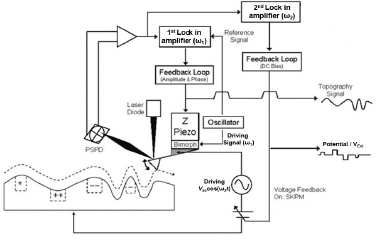Figure 2. KPFM schematic used by Park Systems. Figure 3(e) is the line profile data of the mean values for 16 adjacent points along the y-axis. It can be observed that the metal regions in the potential difference (VExt) image are mutually inverted. This proves that the inverted signs, positive and negative expressions in Equations 2.8 and 2.9 are correct.

The KPFM mode in AFM can be measured in several different ways. One of the methods applied by Park Systems makes use of two frequencies, as shown in Figure 2. Two implemented lock-in amplifiers in the controller are used for each frequency moderation.

One frequency is used to oscillate the cantilever and obtain a surface image using bimorph. Bimorph is a term used for oscillating the cantilever that makes use of piezoelectric material.

The other frequency directly signals the cantilever at 17.0 kHz, which is the frequency generally used for KPFM.

Each frequency provides a topography signal and a potential signal, which creates two images that are independent from one another. Therefore, a single scan allows the user to acquire a surface image and a potential image. The topography signal is obtained by maintaining a constant distance between the tip and sample, whereas the potential image is obtained by applying a default external voltage and potential measurement voltage on the cantilever as described in Figure 2.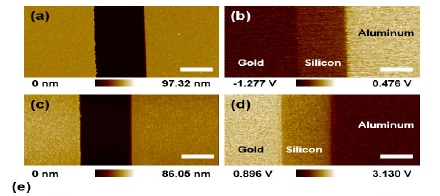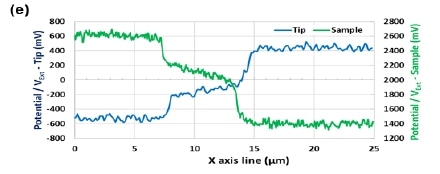Figure 3. 25.0 um x 12.5 um KPFM images of the Au-Si-Al patterned sample. When applied to cantilever direction topography (a) and VExt (b). When applied to sample direction topography (c) and VExt (d). Scale bar is 5 um. (e) is a VExt (potential difference) line profile according to the applied direction.

NCHAucantilever of NANOSENSORS was used for KPFM measurement. This model has a metallic layer coated on both sides of the cantilever and possesses a typical tip radius of curvature, which is smaller than 50 nm.

The resonant frequency and force constant is 330 kHz and 42 N/m, respectively. Figure 3 (e) shows the offset between the tip and the surface sample that may sometimes occur. A possible reason for this offset is the electrical factors that come from the VA amplitude. Hence, one has to measure the HOPG or a calibration sample to find out the offset on the KPFM measurement. The work function values of the samples are known in advance. An important point to note is that the difference between the Au and Al areas must be constant, according to the applied direction, as there is an absolute difference in work function.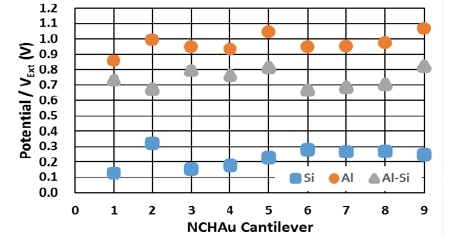Figure 4. KPFM data obtained using nine different NCHAu (NANOSENSORS) probes on the Au-Si-Al patterned sample. The value on Al (orangecircles), Si (blue squares) and their differences (gray triangles) are plotted.

Table 1. Theoretical work function values and nine different NCHAu probes work function values for average for each of the three materials in the Au-Si-Al patterned sample. Park Systems offers a suite of KPFM measurement solutions that serve as a quantitative work function measurement on various samples.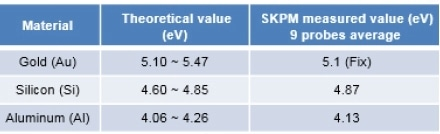### Analysis & Repeatability of KPFM

The main aim of KPFM is to obtain the work function that helps to measure the specimen. The VCPD between the tip and the sample is secondary. Precise analysis is crucial in order to acquire the exact work function of the sample. It is also necessary to measure various cantilevers and find their average. This allows researchers to obtain more concrete work function values of different samples.

Calibration of the system must be carried out using a sample that has a work function that is already known (for instance, HOPG)

First, the measurement of the tip’s work function is conveyed using the sample with a given function. This is done as it limits the electrical offset that could potentially occur during KPFM measurement.

After that, the work function of the tip is obtained using Equation 2.10. Finally, repeating this process several times and averaging the results will produce highly accurate results.

The sample consisted of three different materials: Au, Si, and Al. Au was selected as a base material in order to calibrate the surface sample. The work function of Si and Al were determined as explained above. The theoretical work function values, as well as the experimentally determined work function values of Si and Al, are shown in Table 1.

1. M. Nonnenmacher, M.P. Oboyle, H.K. Wickramasinghe, Appl. Phys. Lett. 58(1991) 2921.
2. H. Hoppe, T. Glatzel, M. Niggemann, A. Hinsch, M.C. Lux-Steiner, N.S. Sariciftci,
3. Nano Lett. 5 (2005) 269.
4. T. Hallam, C.M. Duffy, T. Minakata, M. Ando, H. Sirringhaus, Nanotechnology 20 (2009) 025203.
5. L.M. Liu, G.Y. Li, Appl. Phys. Lett. 96 (2010) 083302.
6. R. Shikler, T. Meoded, N. Fried, B. Mishori, Y. Rosenwaks, J. Appl. Phys. 86 (1999) 107.
7. S.V. Kalinin, A. Gruverman (Eds.), Scanning Probe Microscopy, Springer, New York, 2007.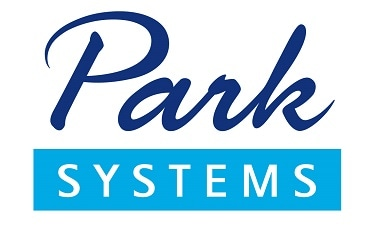This information has been sourced, reviewed and adapted from materials provided by Park Systems Inc.

## Citations

• APA

Park Systems. (2019, November 22). Analyzing Semiconductor Device Surfaces and Electrical Properties. AZoNano. Retrieved on August 06, 2020 from https://www.azonano.com/article.aspx?ArticleID=5057.

• MLA

Park Systems. "Analyzing Semiconductor Device Surfaces and Electrical Properties". AZoNano. 06 August 2020. <https://www.azonano.com/article.aspx?ArticleID=5057>.

• Chicago

Park Systems. "Analyzing Semiconductor Device Surfaces and Electrical Properties". AZoNano. https://www.azonano.com/article.aspx?ArticleID=5057. (accessed August 06, 2020).

• Harvard

Park Systems. 2019. Analyzing Semiconductor Device Surfaces and Electrical Properties. AZoNano, viewed 06 August 2020, https://www.azonano.com/article.aspx?ArticleID=5057.